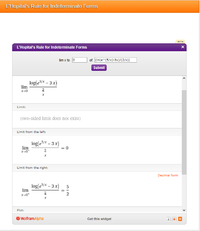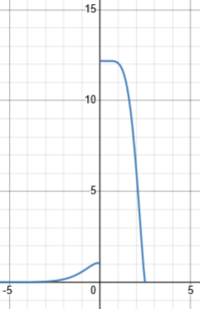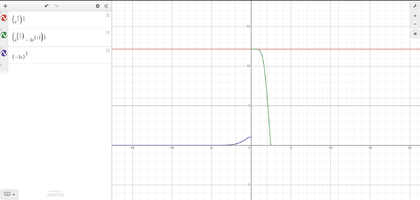# L'Hopital's Rule Problem Help

#### Bzombee

##### New member
Doing a worksheet for class and I was given this problem:
--------
Use l'Hopital's rule to find the exact value of
$\lim_{x \to 0} \left(e^{\frac{5}{x}}-3x\right)^{\frac{x}{2}}$ .
--------
This is what I have done so far:
$\lim_{x \to 0}\ln y=\lim_{x \to 0}\ln\left(e^{\frac{5}{x}}-3x\right)^{\frac{x}{2}}$$\lim_{x \to 0}\ln y=\lim_{x \to 0}\frac{x\ln\left(e^{\frac{5}{x}}-3x\right)}{2}$$\lim_{x \to 0}\frac{x\ln\left(e^{\frac{5}{x}}-3x\right)}{2} = 0$$\lim_{x \to 0}\left(e^{\frac{5}{x}}-3x\right)^{\frac{x}{2}} = \lim_{x \to 0}y = \lim_{x \to 0} e^{\ln y} =e^{0} =1$
The answer key to the worksheet says the answer is actually $e^{2.5}$, not 1 which is what I and the rest of my classmates got. Is the answer key wrong or am I missing something. Thanks!

#### MarkFL

##### Super Moderator
Staff member
I do get what they worksheet key shows. You are letting a still indeterminate form go to zero. You did not apply L'Hopital.

•topsquark

#### Bzombee

##### New member
I do get what they worksheet key shows. You are letting a still indeterminate form go to zero. You did not apply L'Hopital.
Is the $e^{\frac{5}{x}}$ still making it go into an indeterminate form? If so, how do I apply L'Hopital's rule or some other method to solve the problem?

#### MarkFL

##### Super Moderator
Staff member
Is the $e^{\frac{5}{x}}$ still making it go into an indeterminate form? If so, how do I apply L'Hopital's rule or some other method to solve the problem?

Yes, I would write the expression as:

$$\displaystyle \frac{\ln\left(e^{\frac{5}{x}}-3x\right)}{\frac{2}{x}}$$

Now you have a form to which L'Hopital can be applied.

•topsquark

#### Bzombee

##### New member
Yes, I would write the expression as:

$$\displaystyle \frac{\ln\left(e^{\frac{5}{x}}-3x\right)}{\frac{2}{x}}$$

Now you have a form to which L'Hopital can be applied.
As a matter of fact, I did try and write it like that. However, I thought it wouldn't do any good because if you perform l'hopital's rule you get this:
$\frac{\left(\frac{-\frac{5\mathrm{e}^{\frac{5}{x}}}{x^{2}}-3}{\mathrm{e}^{\frac{5}{x}}-3x}\right)}{\left(-\frac{2}{x^{2}}\right)}$ .
This does not get rid of what is leading to indeterminate form which is the e^(5/x).
Putting it into Wolfram Alpha I got the following:Now this leads me to believe that this question has is flawed because it should have told me to solve from the left or to the right of the limit (which it does not, I double checked).

#### MarkFL

##### Super Moderator
Staff member
I do agree that the problem should have stated from the right. I simplified what you have to:

$$\displaystyle \ln(L)=\lim_{x\to0}\left(\frac{5e^{\frac{5}{x}}+3x^2}{2\left(e^{\frac{5}{x}}-3x\right)}\right)$$

Now, divide numerator and denominator by:

$$\displaystyle e^{\frac{5}{x}}$$...

••topsquark and Steven G

#### Bzombee

##### New member
So I divided both numerator and denominator by e^(5/x) like you said
$\left(\frac{\left(\frac{5e^{\frac{5}{x}}+3x^{2}}{e^{\frac{5}{x}}}\right)}{\left(\frac{2\left(e^{\frac{5}{x}}-3x\right)}{e^{\frac{5}{x}}}\right)}\right)$Unless I didn't do something right, it brings me back to where we started. A left hand limit at 0 and a right hand limit at 2.5.

#### MarkFL

##### Super Moderator
Staff member
Let's assume we are taking the limit from the right. Doing the division I suggested, the expression becomes:

$$\displaystyle \frac{5+3x^2e^{-\frac{5}{x}}}{2\left(1-3xe^{-\frac{5}{x}}\right)}$$

•topsquark

#### Bzombee

##### New member
Let's assume we are taking the limit from the right. Doing the division I suggested, the expression becomes:

$$\displaystyle \frac{5+3x^2e^{-\frac{5}{x}}}{2\left(1-3xe^{-\frac{5}{x}}\right)}$$
And plugging that in to a graphing calculator gives me the limit as x approaches from the right of 0 gives me a definitive value at 2.5.
Okay, I think I pretty much got what I need now. Thank you for your help

#### Dr.Peterson

##### Elite Member
And plugging that in to a graphing calculator gives me the limit as x approaches from the right of 0 gives me a definitive value at 2.5.
Okay, I think I pretty much got what I need now. Thank you for your help
It's interesting to ponder why it behaves so differently for positive and negative x. Have you considered that? (Hint: which term is most important in each limit, left and right?)#### Bzombee

##### New member
It's interesting to ponder why it behaves so differently for positive and negative x. Have you considered that? (Hint: which term is most important in each limit, left and right?)

View attachment 35274
When you say positive and negative x, I'm assuming you are referring to the limits? But I did play around with the graphs and I got this which was interesting and I don't know if it was what you are referring to: